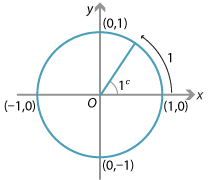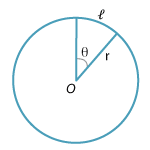## Content

### Review of radian measure

We saw in the module Trigonometric functions and circular measure that angles can be naturally defined using arc length.

We define 1 radian (written as $$1^c$$) to be the angle subtended in the unit circle by an arc length of one unit.Detailed description

Since the circumference of the unit circle is $$2\pi$$ and the angle in one revolution is $$360^\circ$$, we can relate the two units by $$2\pi^c = 360^\circ$$ or

$\pi = 180^\circ.$ (As usual, we will drop the superscript $$c$$ when it is clear that the angle under discussion is in radians.) Many commonly occurring angles can be expressed in radians as fractions of $$\pi$$. For example, $$60^\circ = \dfrac{\pi}{3}$$ and $$330^\circ = \dfrac{11\pi}{6}$$.Detailed description

We have also seen that the arc length $$\ell$$ of a sector of a circle of radius $$r$$, containing an angle $$\theta$$ (in radians), is given by

$\ell = r\theta,$

while the area $$A$$ of the sector is given by

$A = \dfrac{1}{2}r^2\theta.$

Next page - Content - An important limit